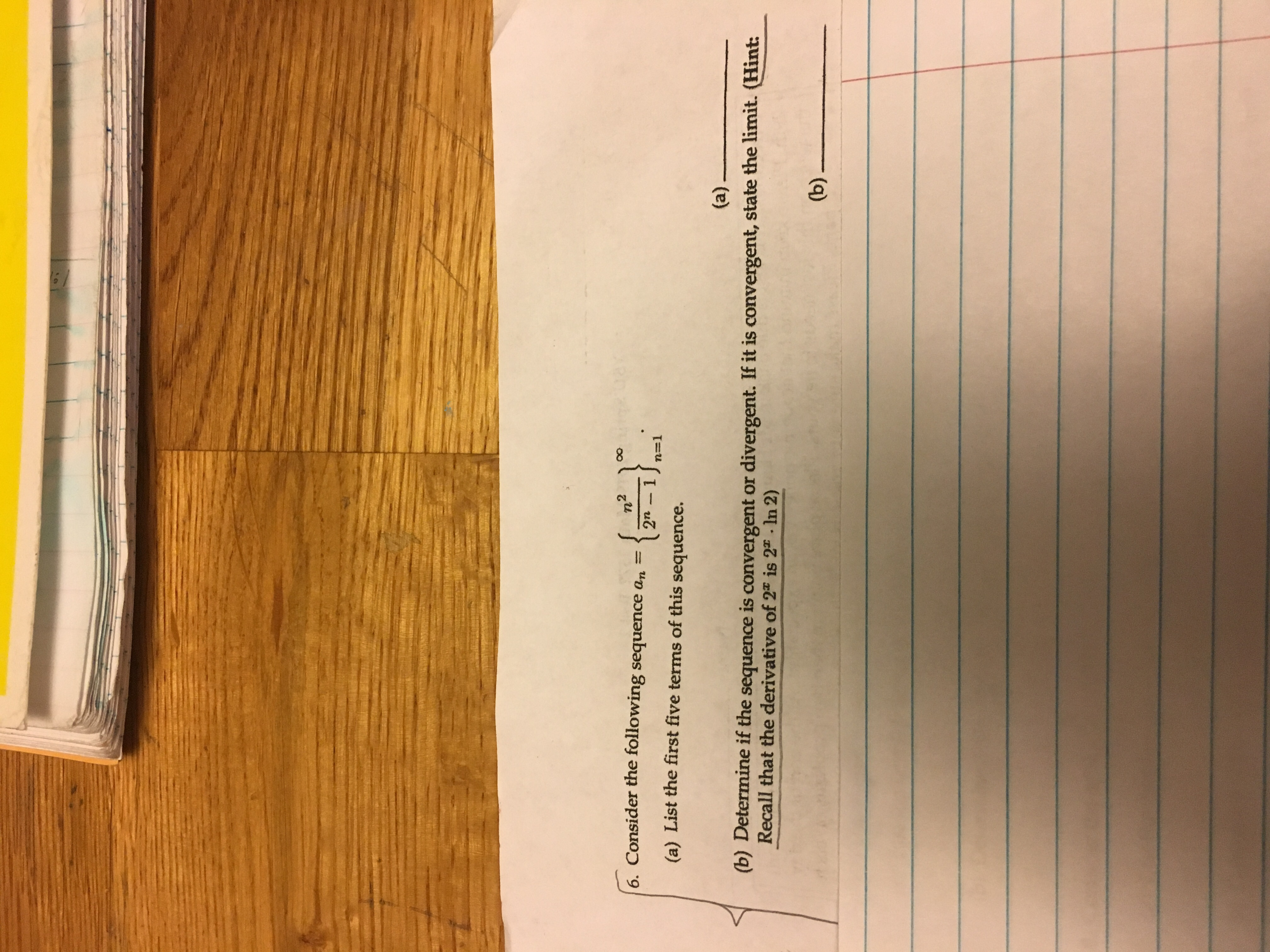# onsider the following sequence an- rn (a) List the first five terms of this sequence. (b) Determine if the sequence is convergent or divergent. If it is convergent, state the limit. (Hint: Recall that the derivative of 2" is 2 In 2)

Questionhelp_outlineImage Transcriptioncloseonsider the following sequence an- rn (a) List the first five terms of this sequence. (b) Determine if the sequence is convergent or divergent. If it is convergent, state the limit. (Hint: Recall that the derivative of 2" is 2 In 2) fullscreen

### Want to see this answer and more?

Experts are waiting 24/7 to provide step-by-step solutions in as fast as 30 minutes!*

*Response times vary by subject and question complexity. Median response time is 34 minutes and may be longer for new subjects.
Tagged in
MathCalculus

### Other